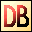DelphiBasicsPredFunction Decrement an ordinal variable System unit
 function Pred ( const Ordinal Value ) : Ordinal type;
Description
The Pred function decrements an ordinal value, and returns this value.

You can decrement :

 Characters Non-floating number types Enumeration types Pointers

The decrement is by the base size of the unit. For example, decrementing a Pointer will be by 2 bytes if the pointer points to Words.

Notes
Pred is equivalent in performance to simple subtraction, or the Dec procedure.
Related commands
 Dec Decrement an ordinal variable Inc Increment an ordinal variable Sqr Gives the square of a number Sqrt Gives the square root of a number Succ Increment an ordinal variable Sum Return the sum of an array of floating point valuesDownload this web site as a Windows program.